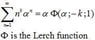# Can this Recursive Solution to an Infinite Sum be Simplified?

aodesky
Consider the infinite sum:

$\sum_{n=1}^\infty \frac{n^2}{2^n}$

For the impatient of you, the answer is here.

Anyways, I'm trying to generalize this result, so let me state a definition:

$\sigma_\alpha(k) = \sum_{n=1}^\infty n^k \alpha ^ n$

This sum converges so long as the magnitude of $\alpha$ is less than 1. I won't prove that; moreover (and I won't bother with the derivation because it involves typing too much LaTeX on my part) I found a way to get solutions to this:

$\sigma_\alpha(k) = \left(\frac{\alpha}{1-\alpha}\right)\left[1 + \sum_{l=0}^{k-1} \binom {k}{l} \sigma_\alpha(l)\right]$

Let's take the cleanest case, where $\alpha={1/2}$.

$\sigma_{1/2}(k) = 1 + \sum_{l=0}^{k-1} \binom {k}{l} \sigma_{1/2}(l)$

Then $\sigma_{1/2}(2)$ should give us the answer above, and it does.

So my question: is there a way to go from my recursive expression for $\sigma_{1/2}(k)$ to a non-recursive formula, just in terms of k?

It would be much easier if there wasn't the "1 + "...

Cheers,
Andrew.

Homework Helper
Hm, how did you arrive at your recursive relation? I'm not sure if the alternate approach I'm about to give is easier or harder, but maybe it will be useful for you. Manipulating your original sum:

$$\sigma_z(k) = \sum_{n=1}^\infty n^k z^n = \sum_{n=1}^\infty \left(z\frac{d}{dz}\right)^k z^n = \left(z\frac{d}{dz}\right)^k\left[\sum_{n=1}^\infty z^n\right] = \left(z\frac{d}{dz}\right)^k\left[\frac{1}{1-z} - 1\right].$$

I assumed, of course, that the order of the sum and the derivative could be swapped, and I wrote z instead of \alpha because I don't want to keep writing \alpha.

Doing the first few derivatives suggests a pattern, which we can use to write (for k > 0)

$$\sigma_z(k) = \sum_{\ell = 1}^k A_\ell(k) \frac{z^\ell}{(1-z)^{\ell+1}};$$
the ##A_\ell(k)## coefficients do not appear to be trivial to sort out. It looks like ##A_1(k) = 1##, ##A_2(k) = 2^{k-1}## and ##A_k(k) = k!##, but inbetween they get tricky. If you can spot a pattern for the ##A_\ell(k)##'s and write down a formula for them, then you could formally prove this sum using induction.

I suspect the recursive form could be turned into this one and vice versa, so maybe you can use that to figure out the ##A_\ell(k)##. The sum may not be doable in closed form, but it won't be recursive. Of course, perhaps you've tried this already before arriving at your recursive form and it won't be of much help.

Millennial
Why don't you simply differentiate the geometric series k times? Consider the argument of the function as alpha instead of k, and differentiate k times with respect to alpha. It should give you a solution, since we know the general form of the geometric series.

JJacquelin

#### Attachments

•Lerch.JPG
3.7 KB · Views: 340
aodesky
Thanks for the replies, everyone.

To Mute:

This should explain where I got the recursive formula.

$$\sigma_z(k) = \sum_{n=1}^\infty n^k z^n = z + 2^k z^2 + 3^k z^3 + ... \\ = (z + z^2 + z^3 + ...) + ((2^k - 1)z^2 + (3^k -1)z^3 + ... ) \\ = \sum_{n=1}^\infty z^n + z\left[ \sum_{n=1}^\infty (n+1)^k z^n - \sum_{n=1}^\infty n z^n \right]\\ = \left[\sum_{n=1}^\infty z^n\right](1-z) + z \sum_{n=1}^\infty (n+1)^k z^n \\ = \left[\sum_{n=1}^\infty z^n\right](1-z) + z \sum_{n=1}^\infty \left[\binom{n}{0}n^0 z^n + \binom{n}{1}n^1 z^n \binom{n}{2}n^2 z^n + ...\right] \\ = z + z \sum_{l=1}^{k-1} \binom{k}{l} \sigma_z(l) + z \sigma_z(k)$$

One step further leads to the relation I originally gave:

$\sigma_\alpha(k) = \left(\frac{\alpha}{1-\alpha}\right)\left[1 + \sum_{l=0}^{k-1} \binom {k}{l} \sigma_\alpha(l)\right]$

To Millennial:

What Mute suggested was essentially what you were getting at. It was an idea that I didn't even consider in either case. It's interesting just to see the conclusion though, for the case k = 2. Using Mute's formula:

$$\sigma_z(k) = \sum_{\ell = 1}^k A_\ell(k) \frac{z^\ell}{(1-z)^{\ell+1}}\\ = A_1(2) \frac{z}{(1-z)^2} + A_2(2) \frac{z^2}{(1-z)^3}\\ = \frac{z}{1-z}\left[A_1(2) \frac{1}{1-z} + A_2(2) \frac{z}{(1-z)^2}\right]\\ = \frac{z}{1-z}\left[1 + A_1(2) \left(\frac{1}{1-z} - 1\right) + A_2(2) \frac{z}{(1-z)^2}\right]$$ (where above I used the fact ##A_1(2)=1##)$$\\ = \frac{z}{1-z}\left[1 + \sigma_z(0) + 2 \sigma_z(1)\right]$$

And finally to JJacquelin:

I see how the Lerch function reduces to this sum in the particular case, I didn't know that it was "famous" haha. It seems to me all they did though is rename the sum? I didn't see an actual solution to the general sum and so it doesn't seem to be in closed form the way I understand it, but definitely interesting to know.

JJacquelin
I see how the Lerch function reduces to this sum in the particular case, I didn't know that it was "famous" haha. It seems to me all they did though is rename the sum? I didn't see an actual solution to the general sum and so it doesn't seem to be in closed form the way I understand it, but definitely interesting to know.
Hello aodesky !
What you say could be said for any special function and more. For example :
$\sum_{n=0}^{∞}x^n/n! = exp(x)$ "all it does though is rename the sum ? "
However, the exp function is very usefull because there is a wide background behind it.
That is why the special functions are so usefull : there is a lot of knowledge gathered about each one. It is also why the Lerch function is usefull: it can be used by people knowing its properties as well as the exp function is used by people knowing its properties.
To French readers : The matter is discussed in the paper "Safari au pays des fonctions spéciales" : http://www.scribd.com/JJacquelin/documents